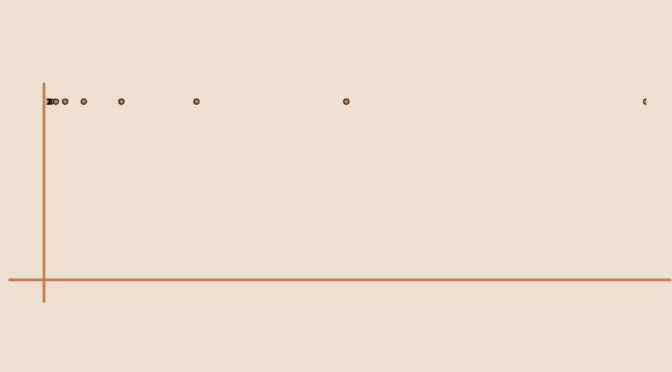# A Riemann-integrable map that is not regulated

For a Banach space $$X$$, a function $$f : [a,b] \to X$$ is said to be regulated if there exists a sequence of step functions $$\varphi_n : [a,b] \to X$$ converging uniformly to $$f$$.

One can prove that a regulated function $$f : [a,b] \to X$$ is Riemann-integrable. Is the converse true? The answer is negative and we provide below an example of a Riemann-integrable real function that is not regulated. Let’s first prove following theorem.

THEOREM A bounded function $$f : [a,b] \to \mathbb R$$ that is (Riemann) integrable on all intervals $$[c, b]$$ with $$a < c < b$$ is integrable on $$[a,b]$$.

PROOF Take $$M > 0$$ such that for all $$x \in [a,b]$$ we have $$\vert f(x) \vert < M$$. For $$\epsilon > 0$$, denote $$c = \inf(a + \frac{\epsilon}{4M},b + \frac{b-a}{2})$$. As $$f$$ is supposed to be integrable on $$[c,b]$$, one can find a partition $$P$$: $$c=x_1 < x_2 < \dots < x_n =b$$ such that $$0 \le U(f,P) - L(f,P) < \frac{\epsilon}{2}$$ where $$L(f,P),U(f,P)$$ are the lower and upper Darboux sums. For the partition $$P^\prime$$: $$a= x_0 < c=x_1 < x_2 < \dots < x_n =b$$, we have \begin{aligned} 0 \le U(f,P^\prime) - L(f,P^\prime) &\le 2M(c-a) + \left(U(f,P) - L(f,P)\right)\\ &< 2M \frac{\epsilon}{4M} + \frac{\epsilon}{2} = \epsilon \end{aligned} We now prove that the function $$f : [0,1] \to [0,1]$$ defined by $f(x)=\begin{cases} 1 &\text{ if } x \in \{2^{-k} \ ; \ k \in \mathbb N\}\\ 0 &\text{otherwise} \end{cases}$ is Riemann-integrable (that follows from above theorem) and not regulated. Let's prove it. If $$f$$ was regulated, there would exist a step function $$g$$ such that $$\vert f(x)-g(x) \vert < \frac{1}{3}$$ for all $$x \in [0,1]$$. If $$0=x_0 < x_1 < \dots < x_n=1$$ is a partition associated to $$g$$ and $$c_1$$ the value of $$g$$ on the interval $$(0,x_1)$$, we must have $$\vert 1-c_1 \vert < \frac{1}{3}$$ as $$f$$ takes (an infinite number of times) the value $$1$$ on $$(0,x_1)$$. But $$f$$ also takes (an infinite number of times) the value $$0$$ on $$(0,x_1)$$. Hence we must have $$\vert c_1 \vert < \frac{1}{3}$$. We get a contradiction as those two inequalities are not compatible.